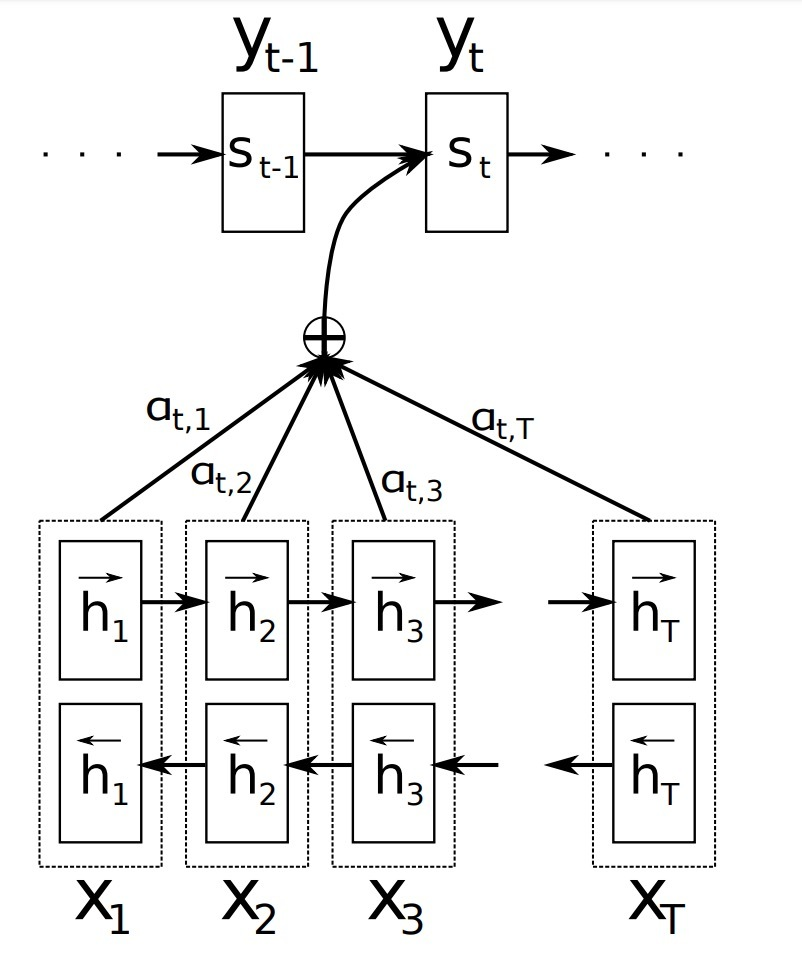attention介绍

April 22, 2016

attention机制在2014年被引入到NLP中：《NEURAL MACHINE TRANSLATION BY JOINTLY LEARNING TO ALIGN AND TRANSLATE》，我们可以看下具体实现：

2.背景：神经机器翻译

2.1 RNN encoder-decoder

$h_t = f(x_t, h_{t-1})$

…(1)

$c = q(\lbrace h_1, \cdots, h_{T_x}\rbrace)$

…(2)

• $h_t \in R^n$是一个在时间t时的hidden state
• **c是一个从hidden states序列生成的vector。
• f和q是一些非线性函数。例如：Suskever 2014使用一个LSTM作为f，$q(\lbrace h_1, \cdots, h_{T_x}\rbrace)=h_T$。

decoder通常被训练成：在给定上下文向量（context vector）c、以及之前预测过的词$\lbrace y_1, \cdots, y_{t'-1}\rbrace$的情况下，用来预测下一个词$y_{t'}$。换句话说，decoder定义了一个在翻译y上的概率，它通过将联合概率(joint probability)解耦成顺序条件（ordered conditionals）：

$p(y) = \prod\limits_{t=1}^T p(y_t | \lbrace y_1, \cdots, y_{t-1} \rbrace, c)$

…(2)

$p(y_t | \lbrace y_1, \cdots, y_{t-1}\rbrace, c) = g(y_{t-1}, s_t, c)$

…(3)

• g是一个非线性、可能多层的函数，会输出概率$y_t$，
• $s_t$是RNN的hidden state。

3.学习align和translate

3.1 Decoder: 通用描述

$p(y_i | y_1, \cdots, y_{i-1}, x) = g(y_{i-1}, s_i, c_i)$

…(4)

$s_i = f(s_{i-1}, y_{i-1}, c_i)$$c_i = \sum\limits_{j=1}^{T_x} \alpha_{ij} h_j$

…(5)

$\alpha_{ij} = \frac{exp(e_{ij})}{ \sum\limits_{k=1}^{T_x} exp(e_{ik})}$

$e_{ij} = a(s_{i-1}, h_j)$

3.2 Encoder：对annotating序列使用Bi-RNN

BiRNN包含forward和backward RNN两部分。forward RNN $\overrightarrow{f}$会按从$x_1$到$x_{T_x}$的顺序读取输入序列，并计算一个forward hidden states序列$(\overrightarrow{h}_1, \cdots, \overrightarrow{h}_{T_x})$。backward RNN $\overleftarrow{f}$会以逆序 （即：从$x_{T_x}$到$x_1$的顺序）来读取序列，产生backward hidden state序列$(\overleftarrow{h}_1, \cdots, \overleftarrow{h}_{T_x})$。

参考

Updated on

STAR算法介绍

PLE介绍

Published on March 04, 2021

DTS介绍

Published on January 02, 2021